Contents Articles Behaviors Books Director News Director Web Sites FAQ Games Mailing Lists News Groups Project Examples Reviews Software Tools Useful Web Sites Utilities Xtras

 Don't miss these#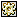Combinations and Permutations

 Compatibilities: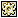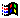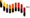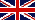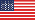This item has not yet been rated

Author: JimAndrews (website)

This movie script calculates C(n,r), P(n,r), and n!. C(n,r) is the number of combinations of n things taken r at a time. P(n,r) is the number of permutations of n things taken r at a time. n! = n(n-1)(n-2)...1.

 --************************************************************************* --C(n,r), P(n,r), and nFactorial(n) --Jim Andrews, August 2004 --vispo.com --This is a movie script. --API: --C(n,r) returns the number of combinations of n things taken r at a time. --See the documentation in the handler itself. --P(n,r) returns the number of permutations of n things taken r at a time. --See the documentation in the handler itself. --nFactorial(n) returns n!=n(n-1)(n-2)...1 --See the documentation in the handler itself. --tester is for running C(n,r) in batch to test it out and see how --it performs in terms of execution time. --************************************************************************* --PUBLIC HANDLERS --************************************************************************* on C(n,r)   --PUBLIC   --PURPOSE************************************************************   --Returns the number of combinations of n things taken r at at time.   --Otherwise known as 'n choose r'. For instance, suppose there are   --five marbles: a red one R, blue one B, green one G, yellow one Y,   --and white one W. Then C(5,2) is the number of combinations of   --5 things taken two at a time. There are 10 such combinations: RB,   --RG, RY, RW, BG, BY, BW, GY, GW, YW. Another example. Suppose   --there are only four marbles and we consider C(4,2). There are   --6 combinations: RG, RB, RW, GB, GW, BW. Note that RG is   --considered to be the same as GB, ie, order does not matter.   --FORMULA*************************************************************   --The formula for C(n,r) = n! / ((n-r)!r!) = n(n-1)...(n-r+1) / r!   --Note that C(n,r)=C(n,n-r)   --PARAMETERS********************************************************   --n is a positive integer. r is also a positive integer and n>=r.   --RETURN VALUE******************************************************   --If n <=0 or r <=0 or n   --this is not the case.   --When C(n,r) <= the maxInteger, C(n,r) returns an integer.   --When C(n,r) > the maxInteger, C(n,r) will either return a float   --or INF if the value exceeds power(10, 308)= 10^308 since   --that is about the largest number Lingo can handle.   --Note that x! gets big very quickly. C(n,r) will return a numeric   --result for any value of n up to 1029 (regardless of the value of r).   --Once n becomes larger than 1029, whether C(n,r) will return a   --numeric value depends on the value of r. Note that for a fixed   --value of n, C(n,r) is maximal for r=n/2.   --Cnr(8000,141)=3.29901039459756e306 but Cnr(8000,142) results in overflow.   --Cnr(9000,137)=3.79579369880744e306 but Cnr(9000,138) results in overflow.   --Cnr(20000,116)=1.75293130532995e308 but Cnr(20000,117) results in overflow.   --Cnr(50000,98)=3.04355441316505e306 but Cnr(50000,99) results in overflow.   --Cnr(200000,80)=1.66268189754524e305 but Cnr(200000,81) results in overflow.   --Cnr(28000000,50)=7.49562229407397e307 but Cnr(28000000,51) results in overflow.   --Note that Lingo only maintains 15 significant digits in floats, so any numbers longer   --than 15 digits have some loss of total accuracy. They are accurate only in the first 14   --digits.   if n > 0 then     if r >0 then       if n>=r then         --Then proceed.         theResult=Combos(n,r,1)         if theResult <= the maxinteger then           --We return an integer result when possible.           return integer(theResult)         else           --Otherwise the result will be a float           return theResult         end if       else         --Else n        return 0       end if     else       --Else r<=0 and n>0       if r<0 then         return 0       else         --Else r=0 and n>0         return 1       end if     end if   else     --Else n<=0     if n<0 then       return 0     else       --Else n=0       if r=0 then         return 1       else         return 0       end if     end if   end if end on nFactorial(n)   --PUBLIC   --PURPOSE****************************************************   --This returns n! = n(n-1)(n-2)...1   --Note that 0!=1 (by logical convention)   --PARAMETERS************************************************   --n is a positive integer <=170   --Values of n > 170 return INF   --because they exceed power(10,308).   --RETURN VALUE**********************************************   --Returns a floating point number if n<=170; returns INF   --otherwise.   if n=1 then     return 1.0   else     if n=0 then       --0!=1       return 1.0     else       return n * nFactorial(n-1)     end if   end if end on P(n,r)   --PUBLIC   --PURPOSE****************************************************   --This returns P(n,r), the number of permutations of n things   --taken r at a time. The difference between this and C(n,r)   --is that order matters in permutations. So, for instance,   --if there are 4 marbles R, G, B, and Y and we consider   --P(4,2), it will be 12 because there are 12 permutations: RG,   --GR, RB, BR, RY, YR, GB, BG, GY, YG, BY, YB   --FORMULA****************************************************   --The formula for P(n,r) = n! / (n-r)! = n(n-1)...(n-r+1)   --PARAMETERS************************************************   --P(n,r) will return a value when n<=170 regardless of what r is.   --When n is larger than 170, P(n,r) will return a value when   --P(n,r) <= power(10,309) and will return INF otherwise.   --because power(10,309) (or thereabouts) is the biggest   --number Lingo can handle.   --RETURN VALUE**********************************************   --Returns a floating point number or INF if the value is too large.   return Permutations(n,r,1) end on tester(n1, n2)   --PUBLIC   --This was written to test the above code.   --This runs C(n,r)   n2 - n1   times.   --For instance, if n1=4 and n2=10, tester will compute   --C(4,2), C(5,2), C(6,3), C(7/3), C(8,4), C(9,4), and C(10,5)   --and display the time each took to compute.   --I suggest you type tester(1,1050) in the message   --window and wait a while for the results.   thetext=""   repeat with i=n1 to n2     n=i     r=i/2     startTime=the milliseconds     theResult=C(n,r)     theEndTime=the milliseconds - startTime     theText=theText & "C(" & string(i) & "," & string(i/2) & ")="  & string(theResult) &  "  time: " & string(theEndTime) & " ms" & RETURN   end repeat   put theText end --************************************************************************* --PRIVATE HANDLERS --************************************************************************* on Combos(n,r, theCount)   --PRIVATE   --This recursive private handler is called by C(n,r) to do the work.   if theCount=r then     return n   else     return Combos(n-1,r, theCount+1) * (float(n)/(r-theCount+1))   end if end on Permutations(n,r, theCount)   --PRIVATE   --This recursive private handler is called by P(n,r) to do the work.   if theCount=r then     return float(n)   else     return n * Permutations(n-1,r, theCount+1)   end if end

 Contact MMI 36 South Court Sq Suite 300 Newnan, GA 30263 USA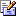Send e-mail